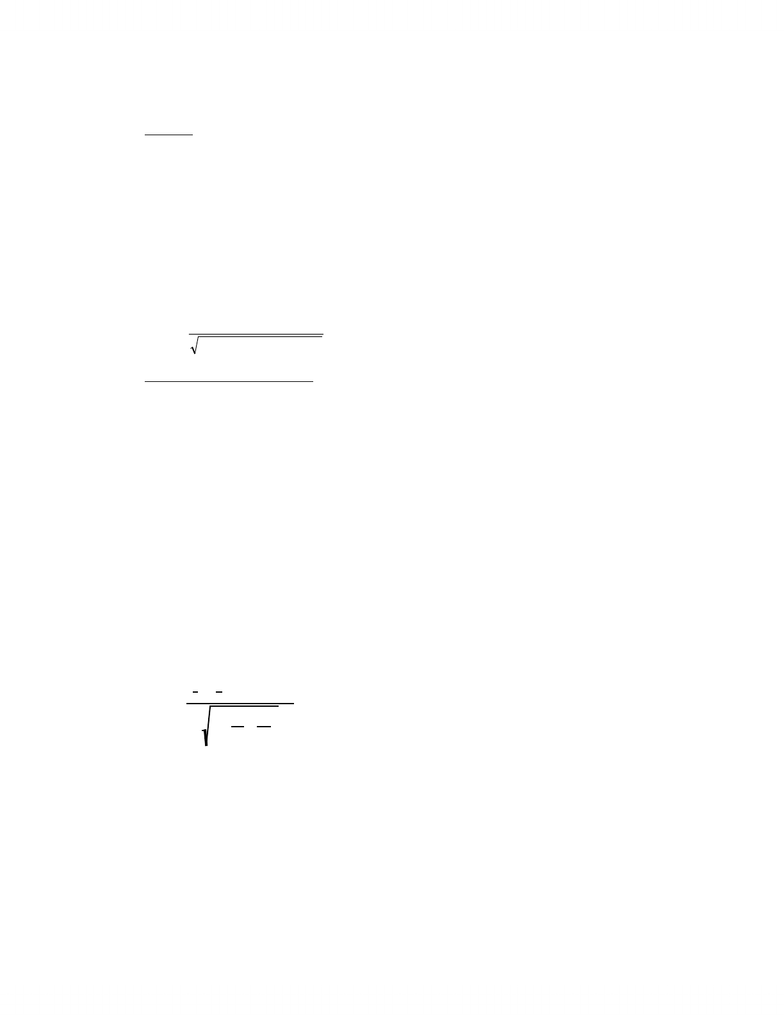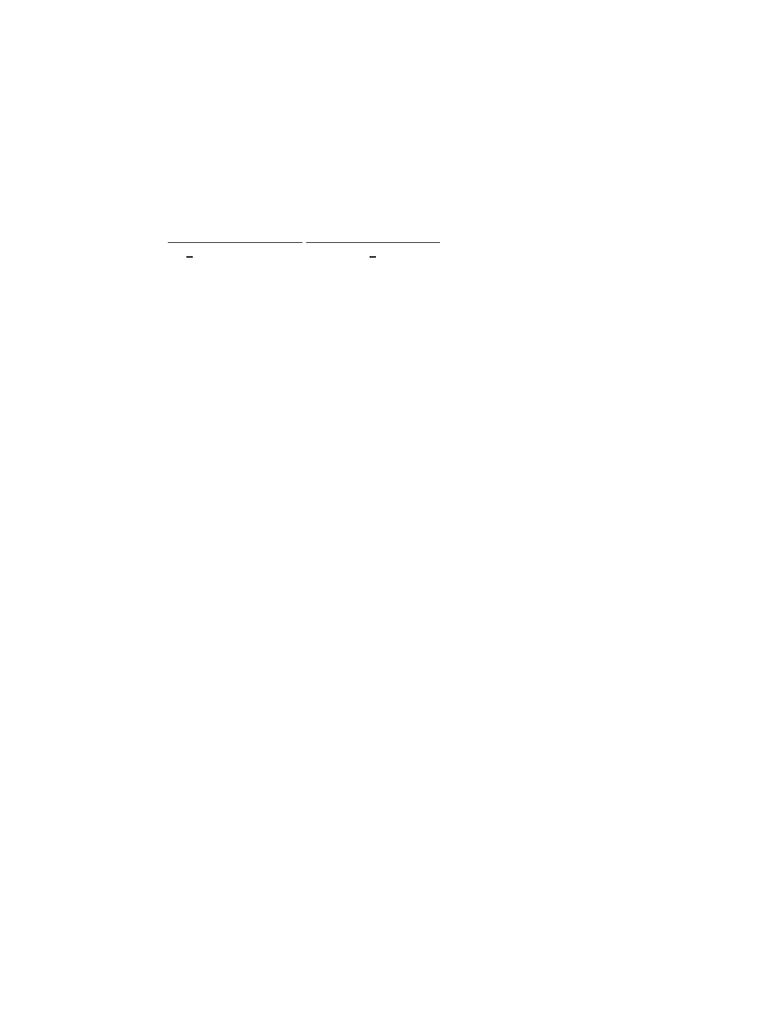Textbook Notes (280,000)
CA (170,000)
York (10,000)
OMIS (50)
Chapter 14

# Chapter 14 Textbook Study Guide

Department
Operations Management and Information System
Course Code
OMIS 2010
Professor
Alan Marshall
Chapter
14

This preview shows pages 1-3. to view the full 9 pages of the document.Chapter 14: Statistical Inference: A Review of Chapters
12 and 13
14.1 Introduction
This chapter was much more than a review of the statistical techniques presented in the previous
three chapters. As we’ve repeatedly said, the most difficult challenge facing statistics students is the
problem of choosing the correct method. At this point, we think it is necessary for you to look back and
check to see whether you can select the appropriate inferential procedure from the 17 already presented.
Obviously, if you cannot identify the right technique now, you will be unable to perform that task later
(in a real-world setting, in another course, or on the final exam in this course) when two or three times as
many methods have been covered.
In this chapter, we provide 13 exercises whose solution requires the use of one of the statistical
techniques introduced in Chapters 12 and 13. We recommend that you use the flowchart exhibited in the
textbook (Figure 14.1). For each exercise, proceed through the flowchart answering all questions in
order to identify the method to use. Then specify the null and alternative hypotheses and the rejection
region (if you're performing calculations manually). Although in our solutions appearing at the back of
this study guide we’ve shown complete solutions, including the calculation of the test statistic, we
suggest that this last step is not absolutely necessary for you. It is far more important that you
concentrate on technique identification than on the simple arithmetic needed to produce the value of the
test statistic. If you’re short of time, do not compute the test statistic.
For an illustration of how the flowchart is to be used, examine the following example.
Example 14.1
Several years ago, two adjacent counties elected school boards with diametrically different philoso-
phies of education. Each school board established its own curriculums and standards. To determine
whether differences exist between the two counties in terms of the success of their high school students,
a preliminary study was launched. Random samples of 100 20-year-olds were drawn from each county.
The respondents were asked the following questions.
a) Did you complete high school?
1. Yes 0. No
b) How many years of high school did you complete?
The data are not shown. (Assume that they were stored on a data disk.)
Determine the statistical techniques and specify the null and alternative hypotheses, the test statistic,
and the rejection region (using ! = 5%) to conclude that differences between the two counties exist in
terms of dropout rates and years of high school completed.
170

Only pages 1-3 are available for preview. Some parts have been intentionally blurred.Solution
For help in answering these questions, we turn to the flowchart.
Dropouts
The first question in the flowchart asks us to determine the problem objective. We want to compare
two populations (the records of the two counties). Next we’re asked to ascertain the type of data. The
data are qualitative, because the responses are “Yes, I completed high school” and “No, I did not com-
plete high school.” The flowchart does not require the answers to any other questions to determine the
correct method to use. It is the z-test of p1
"
p2. The hypotheses and the test statistic are as follows:
H0: = 0
)pp( 21 "
H 1: ! 0
)pp( 21 "
Test statistic:
)n/n/()p
ˆ
(p
ˆ
)p
ˆ
p
ˆ
(
z
21
21
111 #"
"
\$
Years of High School completed
The problem objective is the same—to compare two populations. The data are quantitative, because
in this case we’re working with real numbers that were not arbitrarily assigned—years of high school
completed. For this combination of problem objective and data type, the flowchart has more questions.
The next question asks for the descriptive measurement. We’re interested in comparing the population
locations to determine if one county is more successful than the other in persuading students to stay in
high school. Next we’re asked to identify the experimental design. The experiment did not require any
matching between the two samples. Accordingly, we specify that the samples are independent. Next,
we’re asked whether the data are normal. Drawing histograms of each group would provide some
insight. Let us suppose that the histograms are bell-shaped. The flowchart then asks if the population
variances are equal. We can conduct an F-test of to answer. We’ll assume that they are. At this
point, the flowchart tells us that the appropriate technique is the equal-variances test of µ1 µ2. The test
would be conducted in the following way:
%
1
2/
%
2
2
H0: ( = 0 &1"&
2)
H 1: ( ' 0 &1"&
2)
Test statistic:
t\$(
x
1"
x
2)
"
(&1"&2)
sp
21
n1
#1
n2
()
*)
+) ,)
-)
.)
The computer could be used to produce the values of the test statistic and draw conclusions.
171

Only pages 1-3 are available for preview. Some parts have been intentionally blurred.EXERCISES
14.1 In the game of squash, it is quite common for the ball to attain speeds in excess of 100 mph. As
a result, squash balls do not last very long. In an effort to improve the longevity of squash balls,
a sporting goods manufacturer has been experimenting with a new type of squash ball construc-
tion. To assess the results, 50 squash balls made the conventional way and another 50 made the
experimental way are distributed to amateur squash players. Each player is told to use the
squash balls until they split and record the number of complete games for which the balls were
used. The statistical results are as follows:
Conventional Squash Ball Experimental Squash Ball
x
1 = 13.6 games
x
2 = 14.7 games
s1 = 3.9 games s2 = 1.8 games
Do these statistics allow the manufacturer to conclude at the 5% significance level that the
experimental squash ball is superior?
H0:
H1:
Test statistic:
Rejection region:
Value of the test statistic:
Conclusion:
172